HomeAudienceDevelopersA quick look at integer promotion in C

# A quick look at integer promotion in C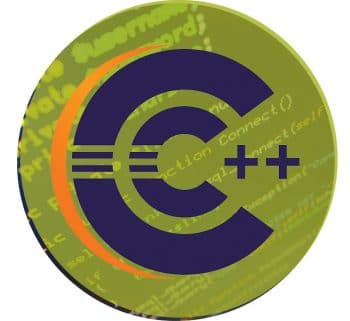Data types like char and short int take a fewer number of bytes than int. These data types are automatically promoted to int or unsigned int when an operation is performed on them. This is known as integer promotion.

We have all heard the popular quote about the C language that C is an ocean, not a sea. No matter how much of knowledge you gain in C, theres always something new to learn about it. In this article, I focus on integer promotion in C.

What is integer promotion in C?
Whenever any operations like arithmetic, bitwise are performed on lower rank data types such as char and short, automatically these data types will be implicitly promoted to a higher rank data type, signed int or unsigned int, until and unless explicitly typecasted.
Let us understand the above statement with the help of the following example, Code-1:

```1. #include <stdio.h>
2. int main()
3. {
4. signed char x = 0xFF;
5. unsigned char y = 0xFF;
6. //Compare both the values of x and y
7. if (x == y)
8. {
9. printf(Equal\n);
10. }
11. else
12. {
13. printf(Unequal\n);
14. }
15. return 0;
16. }```

Code-1: Code to compare two char values of different qualifiers

What will be the output of Code-1? Most people may think the answer is Equal but, in fact, it is Unequal; that is why C is called a tricky language too. The output of Code-1 is shown in Figure 1.

In order to understand why the output is unequal, let us look at one more simple example, Code-2:

```1 #include <stdio.h>
2
3 int main()
4 {
5 signed char x = 0xFF;
6 unsigned char y = 0xFF;
7
8 printf("The value of x: %X\n", x);
9 printf("The value of y: %X\n", y);
10
11 return 0;
12 }```

Code-2: Code to print the values of x and y

Since x is declared as the char data type, one byte of memory will be allocated and 0xFF is the value stored in it. So, printing the value of x using the %X format specifier results in integer type promotion. Also, since the MSB bit is set in this case, when it is promoted, all the bits in the remaining three bytes of the int type will be filled with the value of the MSB bit. Hence, all the bits will be filled with 1, because the MSB bit is 1 in the x variable as shown in Figure 3.
Also, since y is declared as the unsigned char data type, one byte of memory will be allocated and 0xFF is the value stored in it. So, printing the value of x using the %X format specifier will result in integer type promotion. Since the MSB is not reserved for storing the sign of the number when it is promoted, all the bits in the remaining three bytes of the int type will be filled with zeros, irrespective of whether MSB of lower rank data type is SET or CLEAR, as shown in Figure 4.
So from the explanation given for Code-2, it is clear that char is promoted to int data type. Now going back to Code-1, when two variables are compared in the if statement, integer type promotion takes place. As a result, the value of x is converted to 0xFFFFFFFF since x is a signed char, and the value of y is converted to 0x000000FF because y is unsigned char. After implicit type promotion, the values are compared and found unequal. For more clarity, refer to Figure 5.
Let us dig a little deeper by obtaining the assembly code for Code-1 and analysing the disassembled code as shown in Figure 6, the explanation for which is given in Table 1.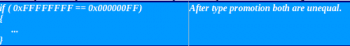Figure 5: Consolidated pictorial representation for Code – 1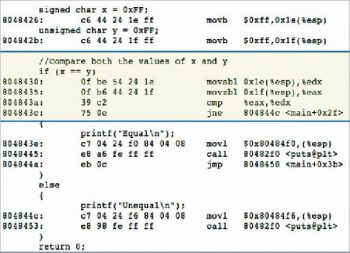Figure 6: Disassembled code for the source Code – 1

Note: In C99, integer promotion is clearly defined in the following rule (6.3.1.1): If an int can represent all values of the original type, the value is converted to an int; otherwise, it is converted to an unsigned int. These are called the integer promotions. All other types are unchanged by the integer promotions.

Let us consider another example (Code-3) to understand integer promotion:

```1 #include <stdio.h>
2 int main()
3 {
4 signed char x, y, z, res;
5 x = 20;
6 y = 30;
7 z = 8;
8 res = x * y / z;
9 printf(Res: %d\n, res);
10 return 0;
11 }```

Code-3: Code to show how integer promotion takes place in an expression that involves arithmetic operators

Consider the expression res = x * y / z; present in Code-3. In this expression, there are three operators (=, *, /). Among these three, the * and / operators have the same precedence; hence, associativity comes into the picture. According to the associativity rule for the arithmetic operators, evaluation is from left to right. So, * will be evaluated first. Before evaluating the sub-expression x * y, both the operands will be type promoted to either int or unsigned int, according to the rules of c99 standards (see the Note above).
The result of x * y is 600 according to the values given in Code-3, which seems to be arithmetic overflow because 600 does not fall in the range -128 to +127 (minimum and maximum limit of the signed char). However, due to integer promotion the operation is smoothly carried out without any overflow.
In the expression, the result of the sub-expression is further divided by z, which is a signed char. As a result, 600, which is in int form, is divided by the value of z, i.e., 8. This yields the result 75, which is in int form. Since 75 falls within the range (-128 to +127), without any side effect, this value is assigned to the res variable after demoting it to signed char.
Table 2 gives the explanation for the disassembled code shown in Figure 9.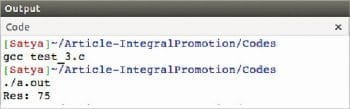Figure 7: Output of Code – 3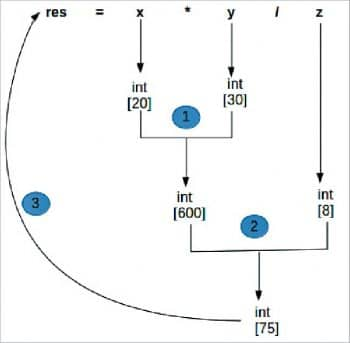Figure 8: Pictorial representation of the type promotion for the expressions = x * y/z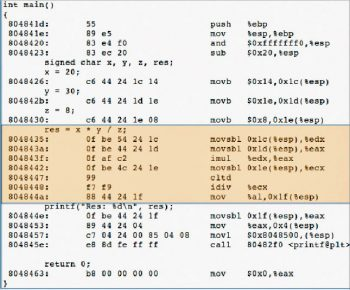Figure 9: Disassembled code for the source Code – 3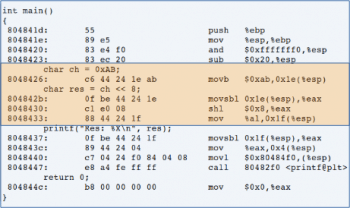Figure 10: Disassembled code for the source Code – 4

Let us consider the last example (Code-4) to understand integer promotion.

Think about whether we can apply, bitwise, the left shift operator on a char data type more than the bits present in the char? For instance, ch << 8, since the maximum left shifting possible is only seven times.

```1 #include <stdio.h>
2 int main()
3 {
4 char ch = 0xAB;
5 char res = ch << 8;
6 printf(Res: %X\n, res);
7 return 0;
8 }```

Code-4: Code to show how integer promotion takes place in a bitwise left-shift operation

The left-shift operator can be applied to the character variable more than the limited size, which is 7, but it is of no use, even though the compiler will not generate any errors. In this case, the output will always be zero. An in-depth explanation can be found about disassembled code in Figure 10 and Table 3.

In order to improve the performance of the operations, the compiler will internally apply the implicit type conversion rules to the data types that have a lower rank than that of the int data type. But the programmer has to take care of overflow issues during the operations. Performing the operations on the natural size of the machine is very fast compared to the other data types whose size is not equal to the natural size of the machine, like char and short. While writing the expressions involving multiple data types, the programmer has to ensure that the expression is free of side effects.